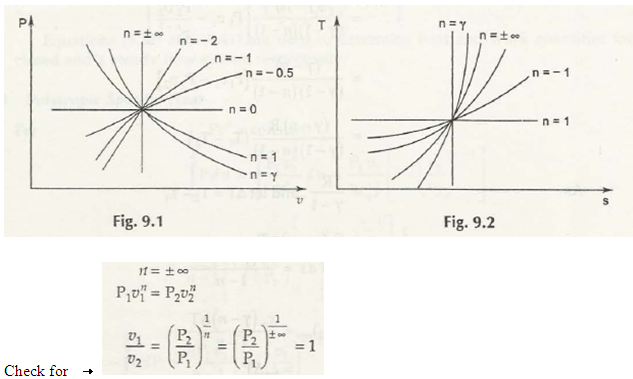V n d P + P n v n 1 dv = 0

dp / du = – n p / u

slope = – n p / u

From there we get that slope depends on P and v for a particular n, in negative sense.

 Process Value of n Equation of process Isobaric ProcessIsothermal ProcessIsentropic ProcessIsometric or isochoric Process n = 0n = 1n = y· P = Const.T = Const.S = Const.v = Const.Similarly u1 = u2

u2 = u3 and so on

u = constant for n=∞

Links of Next Mechanical Engineering Topics:-### Customer Reviews

My Homework Help
Rated 5.0 out of 5 based on 510 customer reviews at